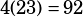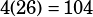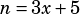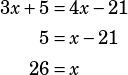Test 3 Section 4 #24

Of course, we could use algebra to solve this (and I will down below), but because the answer choices are numbers that can easily be dropped back into the word problem, my recommended strategy is backsolving.

Start by trying C; assume 23 students in the class. If Mr. Kohl gives each student 3 ml, he’ll have 5 ml left over.. For Mr. Kohl to give each student 4 ml, he’ll need an additional 21 ml.. Is that 21 higher than 74? It is not—it’s only 18 more.

That suggests we need even more kids in the class, so the answer is almost certainly D, which says there are 26 students. If Mr. Kohl gives each student 3 ml, he’ll have 5 ml left over.. For Mr. Kohl to give each student 4 ml, he’ll need an additional 21 ml.. Is that 21 higher than 83? Yes! D is the answer.

Now, the algebra. What we want to do here is come up with two expressions that are both equal to n, the amount of solution Mr. Kohl has. I’ll use x for the number of students he has.

If Mr. Kohl gives each student 3 ml, he’ll have 5 ml left over.In order to give each student 4 ml, he will need an additional 21 ml.Now that we have those equations, we can solve by substitution.